# How to Calculate and Solve for Apparent Conductivity | Electromagnetic Method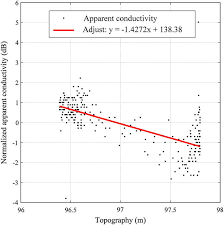The image above represents apparent conductivity.

To compute for apparent conductivity, five essential parameters are needed and these parameters are Angular Velocity (ω), Magnetic Permeability (μo), Coil Spacing (S), Amplitude of Secondary Field (Hsand Amplitude of Primary Field (Hp).

The formula for calculating apparent conductivity:

σ = 4Hs / ωμoS²Hp

Where:

σ = Apparent Conductivity
ω = Angular Velocity
μo = Magnetic Permeability
S = Coil Spacing
Hs = Amplitude of Secondary Field
Hp = Amplitude of Primary Field

Let’s solve an example;
Find the apparent conductivity when the angular velocity is 3, the magnetic permeability is 12, the coil spacing is 9, the amplitude of secondary field is 2 and the amplitude of primary field is 4.

This implies that;

ω = Angular Velocity = 3
μo = Magnetic Permeability = 12
S = Coil Spacing = 9
Hs = Amplitude of Secondary Field = 2
Hp = Amplitude of Primary Field = 4

σ = 4Hs / ωμoS²Hp
σ = 4(2) / 3(12)(9)²(4)
σ = 8 / 3(12)(81)(4)
σ = 8 / 11664
σ = 0.000685

Therefore, the apparent conductivity is 0.000685.

Nickzom Calculator – The Calculator Encyclopedia is capable of calculating the apparent conductivity.

To get the answer and workings of the apparent conductivity using the Nickzom Calculator – The Calculator Encyclopedia. First, you need to obtain the app.

You can get this app via any of these means:

To get access to the professional version via web, you need to register and subscribe for NGN 1,500 per annum to have utter access to all functionalities.
You can also try the demo version via https://www.nickzom.org/calculator

Apple (Paid) – https://itunes.apple.com/us/app/nickzom-calculator/id1331162702?mt=8
Once, you have obtained the calculator encyclopedia app, proceed to the Calculator Map, then click on Geology under Add-on.Now, Click on Electromagnetic Method under GeologyNow, Click on Apparent Conductivity under Electromagnetic Method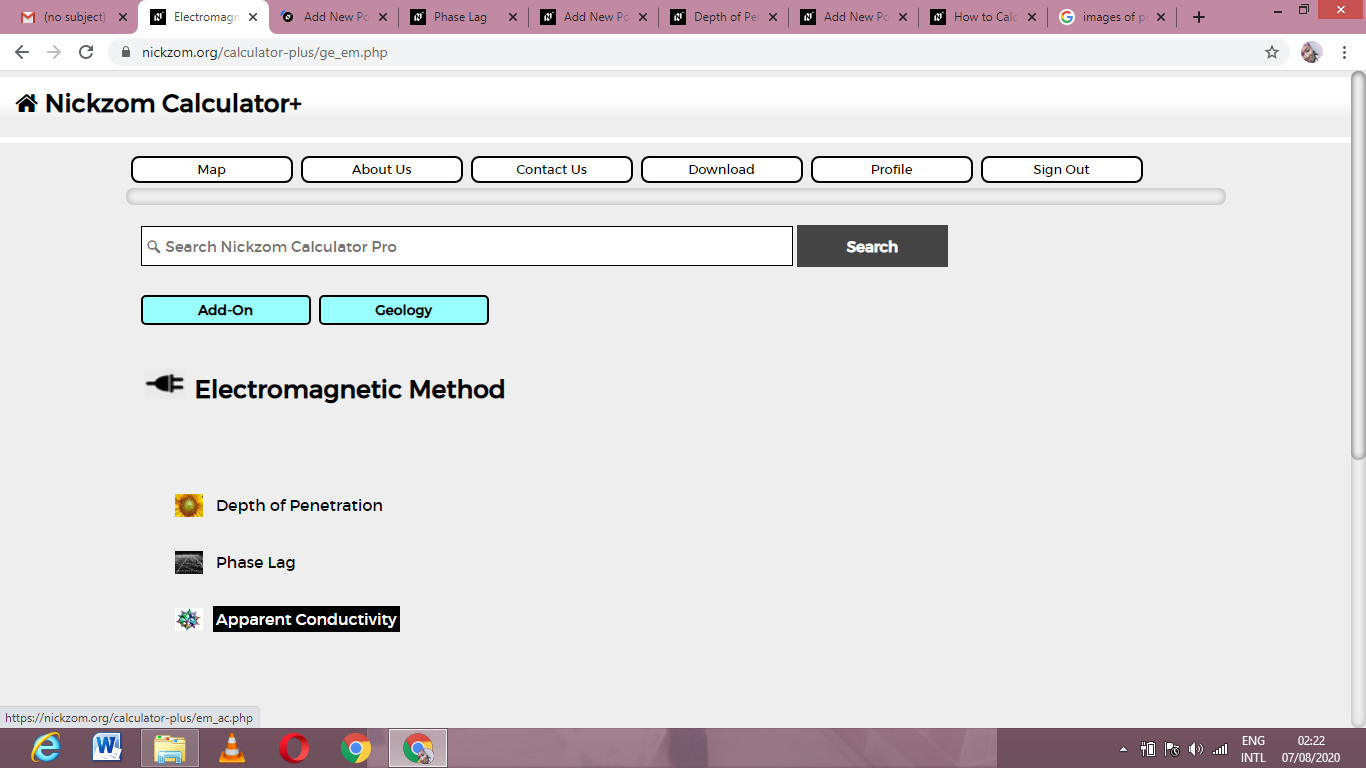The screenshot below displays the page or activity to enter your values, to get the answer for the apparent conductivity according to the respective parameters which are the Angular Velocity (ω), Magnetic Permeability (μo), Coil Spacing (S), Amplitude of Secondary Field (Hsand Amplitude of Primary Field (Hp).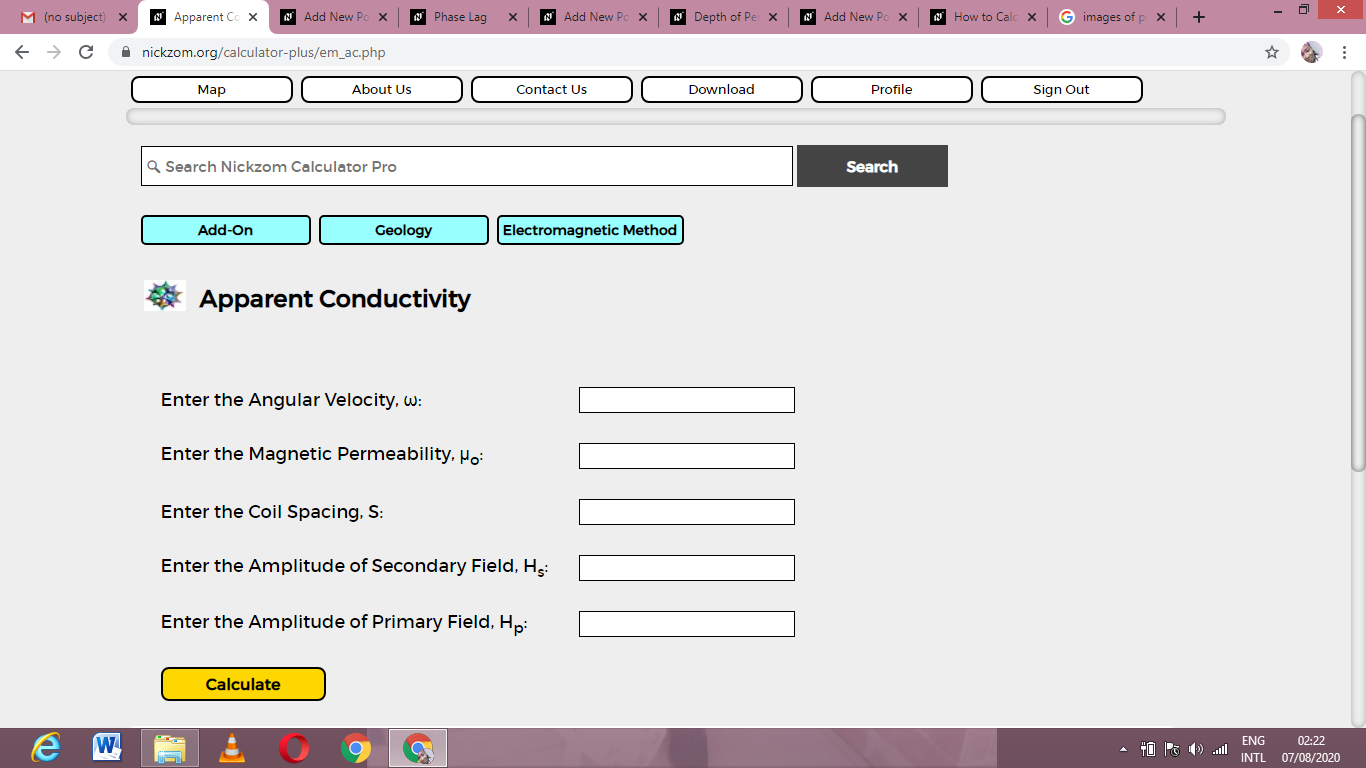Now, enter the values appropriately and accordingly for the parameters as required by the Angular Velocity (ω) is 3, Magnetic Permeability (μo) is 12, Coil Spacing (S) is 9, Amplitude of Secondary Field (Hs) is 2 and Amplitude of Primary Field (Hp) is 4.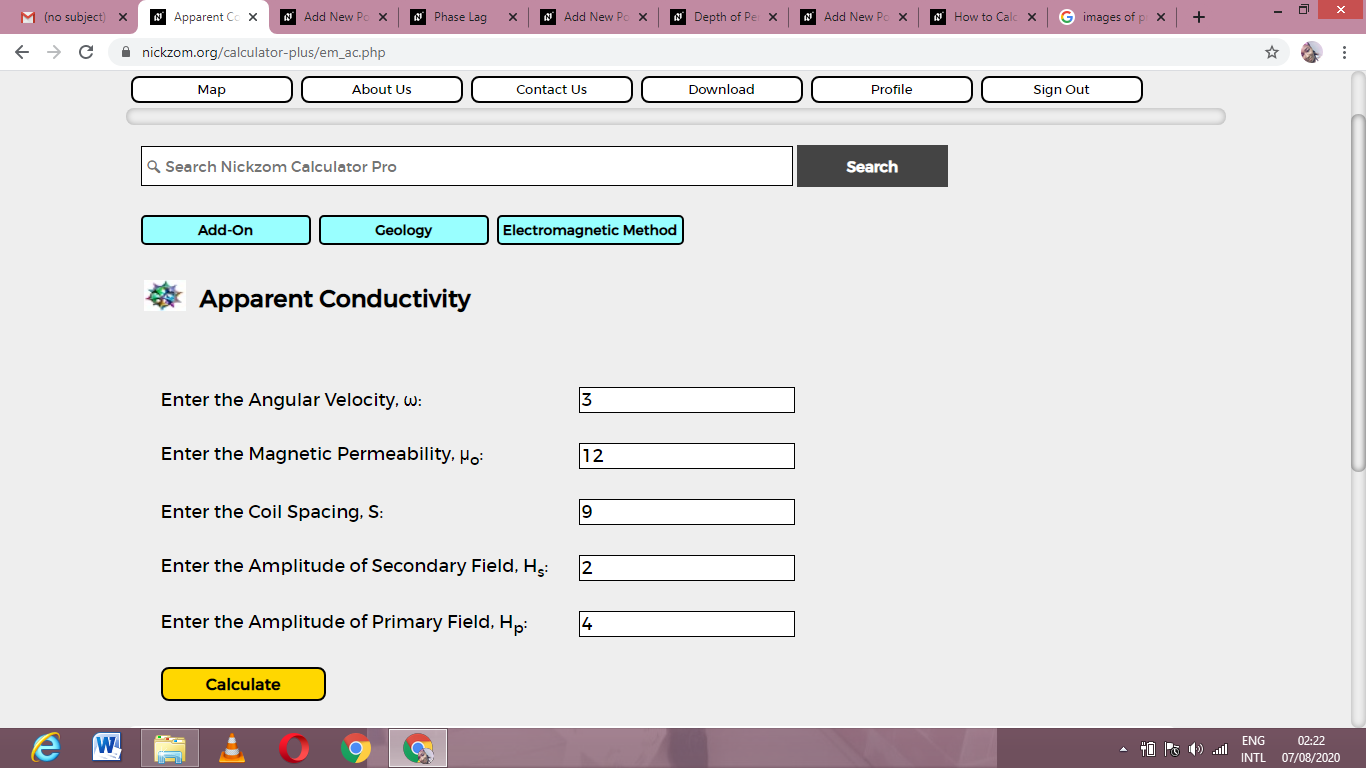Finally, Click on Calculate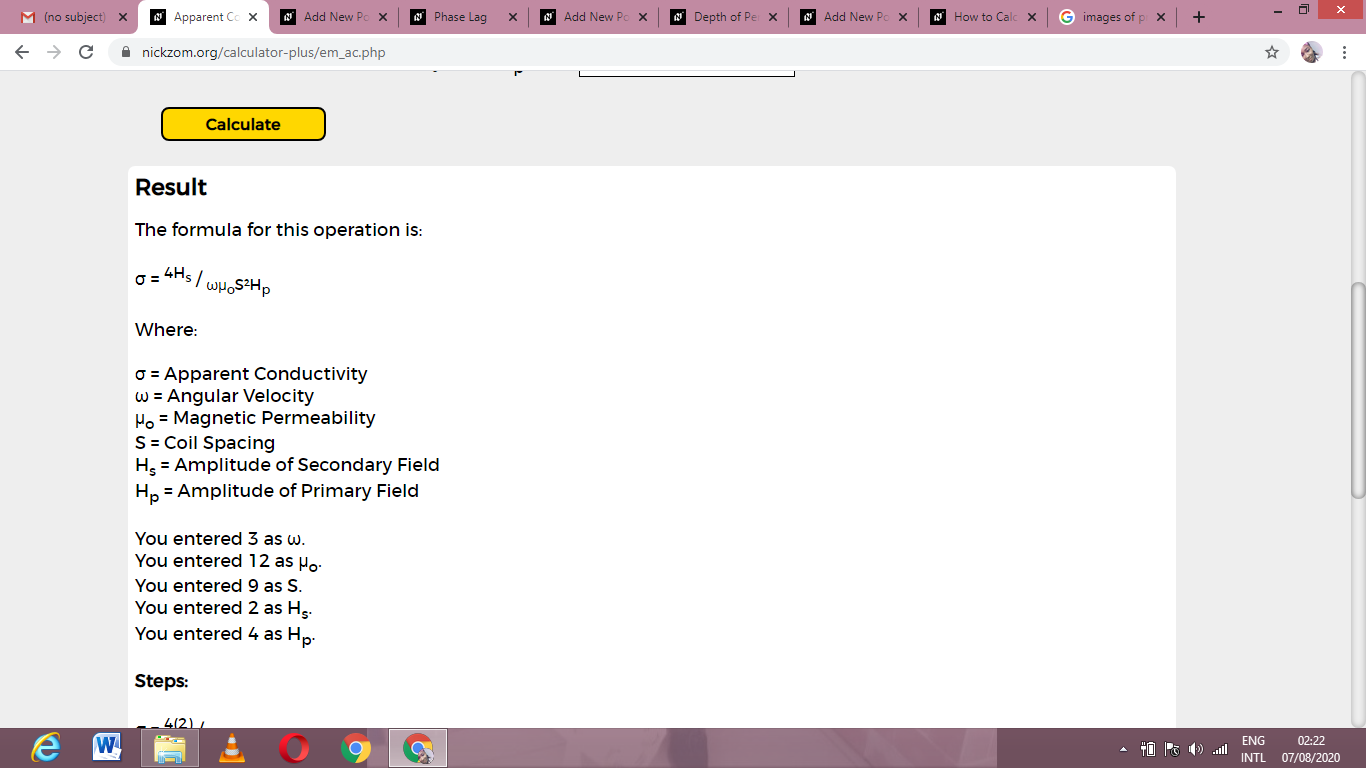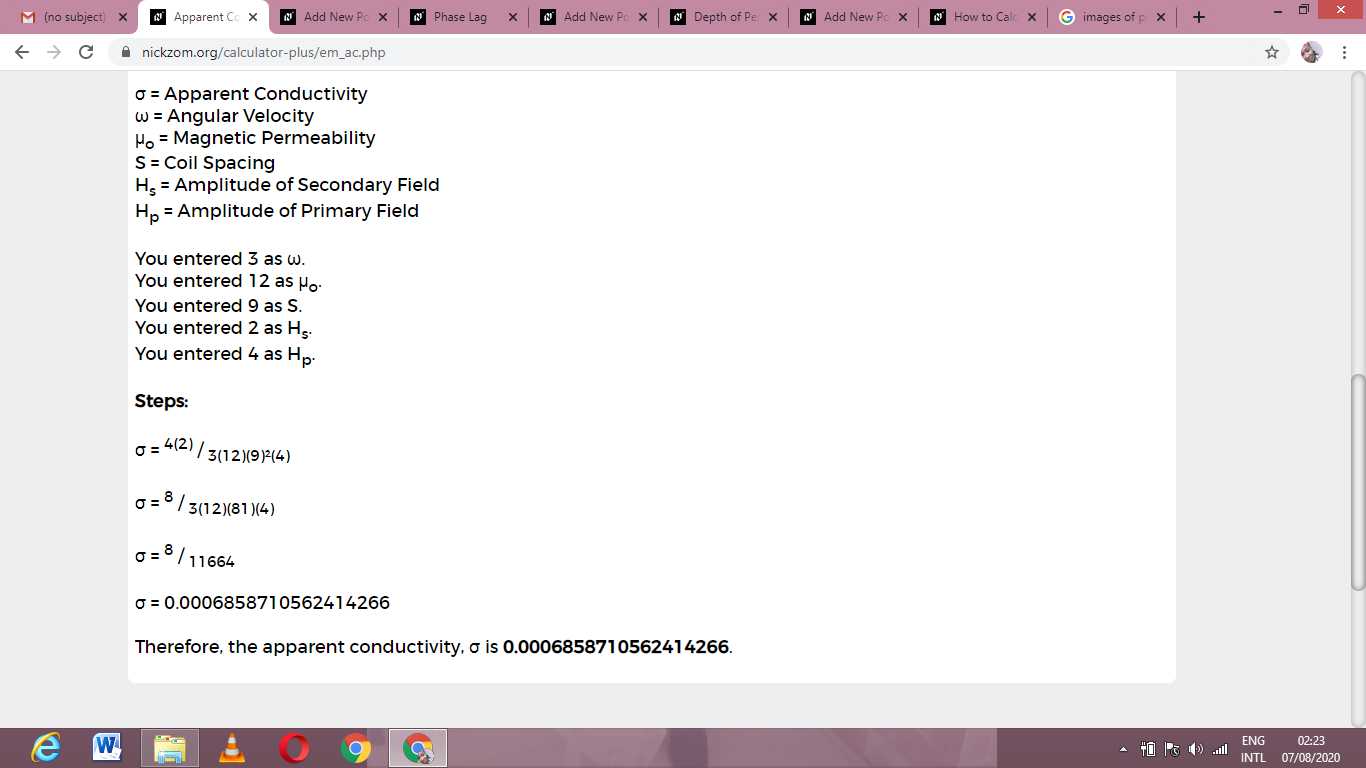As you can see from the screenshot above, Nickzom Calculator– The Calculator Encyclopedia solves for the apparent conductivity and presents the formula, workings and steps too.